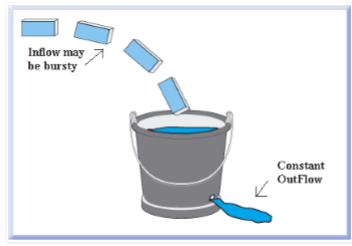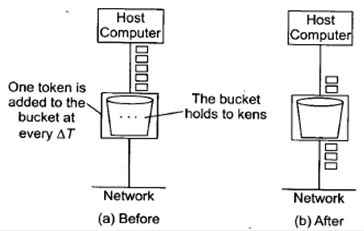Courses

# Congestion Control Computer Science Engineering (CSE) Notes | EduRev

## Computer Science Engineering (CSE) : Congestion Control Computer Science Engineering (CSE) Notes | EduRev

The document Congestion Control Computer Science Engineering (CSE) Notes | EduRev is a part of the Computer Science Engineering (CSE) Course GATE Computer Science Engineering(CSE) 2022 Mock Test Series.
All you need of Computer Science Engineering (CSE) at this link: Computer Science Engineering (CSE)

Congestion Control

What is congestion?

A state occurring in network layer when the message traffic is so heavy that it slows down network response time.

Effects of Congestion

• As delay increases, performance decreases.
• If delay increases, retransmission occurs, making situation worse.

Congestion control algorithms

• Leaky Bucket Algorithm

Let us consider an example to understand

Imagine a bucket with a small hole in the bottom.No matter at what rate water enters the bucket, the outflow is at constant rate.When the bucket is full with water additional water entering spills over the sides and is lost.Similarly, each network interface contains a leaky bucket and the following steps are involved in leaky bucket algorithm:

1.  When host wants to send packet, packet is thrown into the bucket.
2.  The bucket leaks at a constant rate, meaning the network interface transmits packets at a constant rate.
3.  Bursty traffic is converted to a uniform traffic by the leaky bucket.
4. In practice the bucket is a finite queue that outputs at a finite rate.
• Token bucket Algorithm

Need of token bucket Algorithm:-

The leaky bucket algorithm enforces output pattern at the average rate, no matter how bursty the traffic is. So in order to deal with the bursty traffic we need a flexible algorithm so that the data is not lost. One such algorithm is token bucket algorithm.

Steps of this algorithm can be described as follows:

1. In regular intervals tokens are thrown into the bucket. ƒ
2. The bucket has a maximum capacity. ƒ
3. If there is a ready packet, a token is removed from the bucket, and the packet is send.
4. If there is no token in the bucket, the packet cannot be send.

Let’s understand with an example,

In figure (A) we see a bucket holding three tokens, with five packets waiting to be transmitted.For a packet to be transmitted, it must capture and destroy one token. In figure (B) We see that three of the five packets have gotten through, but the other two are stuck waiting for more tokens to be generated.

Let’s understand with an example,Offer running on EduRev: Apply code STAYHOME200 to get INR 200 off on our premium plan EduRev Infinity!

## GATE Computer Science Engineering(CSE) 2022 Mock Test Series

131 docs|167 tests

,

,

,

,

,

,

,

,

,

,

,

,

,

,

,

,

,

,

,

,

,

;